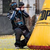0

# Refresh Oxyplot Lineview

Daniel Kampert 5 years ago
Hello,

I want display some Sensordata with Oxyplot. I have this WPF Code:

<oxy:PlotView x:Name="DataPlot" Model="{Binding PlotModel}" Margin="0,127,0,0"/>

My Oxyplot Class

public PlotModel PlotModel {get; private set;}
public int x = 0;
public MainViewModel()
{
this.SetupModel();
}
public void AddDatapoint(int Plot, double x, double y)
{
lock (PlotModel.SyncRoot)
{
var Series = (LineSeries)PlotModel.Series[Plot];

if (Series.Points.Count >= 200)
{
Series.Points.RemoveAt(0);
}

}
}
private void SetupModel()
{
PlotModel = new PlotModel();
PlotModel.Axes.Add(new LinearAxis { Position = AxisPosition.Left, Minimum = -10, Maximum = 10 });
for (int i = 0; i < 20; i++)
{
PlotModel.Series.Add(new LineSeries { LineStyle = LineStyle.Solid });
}
var dispatcherTimer = new System.Windows.Threading.DispatcherTimer { Interval = new TimeSpan(0, 0, 0, 0, 50) };
dispatcherTimer.Tick += RefreshPlot;
dispatcherTimer.Start();
}
private void RefreshPlot(object sender, EventArgs e)
{
{
PlotModel.InvalidatePlot(true);
});
}

Now I have my Mainclass and I want to refresh my Plot. So I use this code:

public Window_Plot()
{
this.DataContext = new MainViewModel();
InitializeComponent();
UpdateTimer = new Timer(Timerupdate);
UpdateTimer.Change(Timeout.Infinite, Timeout.Infinite);
UpdateTimer.Change(1000, 20);
}
public void Timerupdate(object source)
{